Created November 2, 2019 © CC BY-NC-SA

# Autonomous Robot Controlled with Pyro Sensors

Proximity Sensor KEMET used in two applications to avoid crashes: 1) Open and close the gate, and 2) Stop the self driving car

## Things used in this project

### Hardware components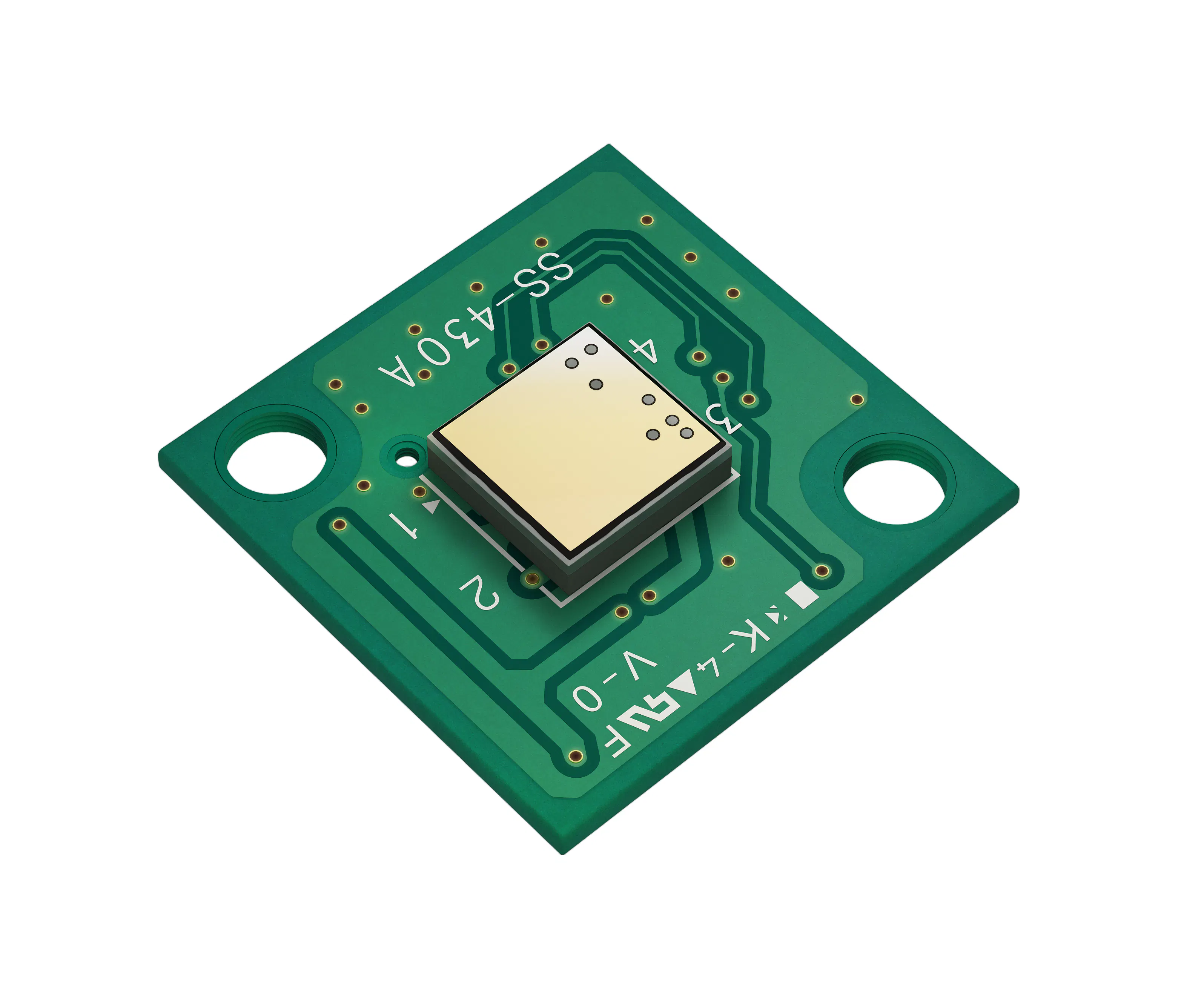KEMET Electronics Corporation Proximity Sensor- Pyroelectric Infrared Sensor Module
×2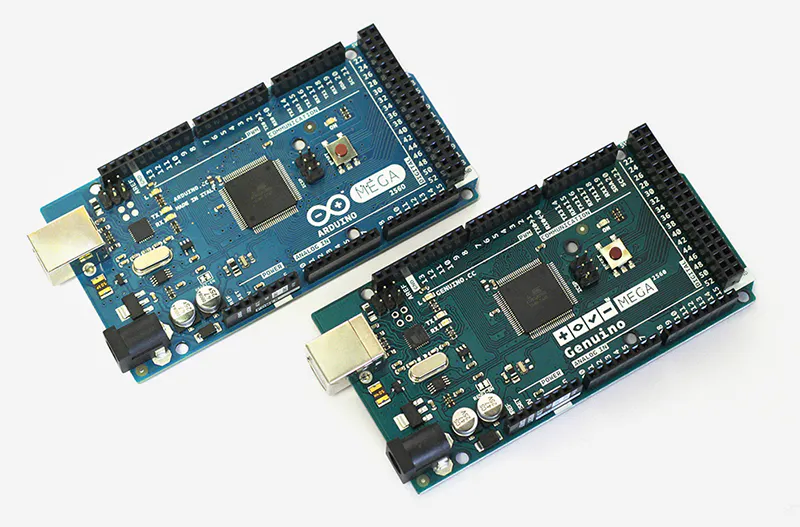Arduino Mega 2560 & Genuino Mega 2560
×1Arduino UNO & Genuino UNO
×1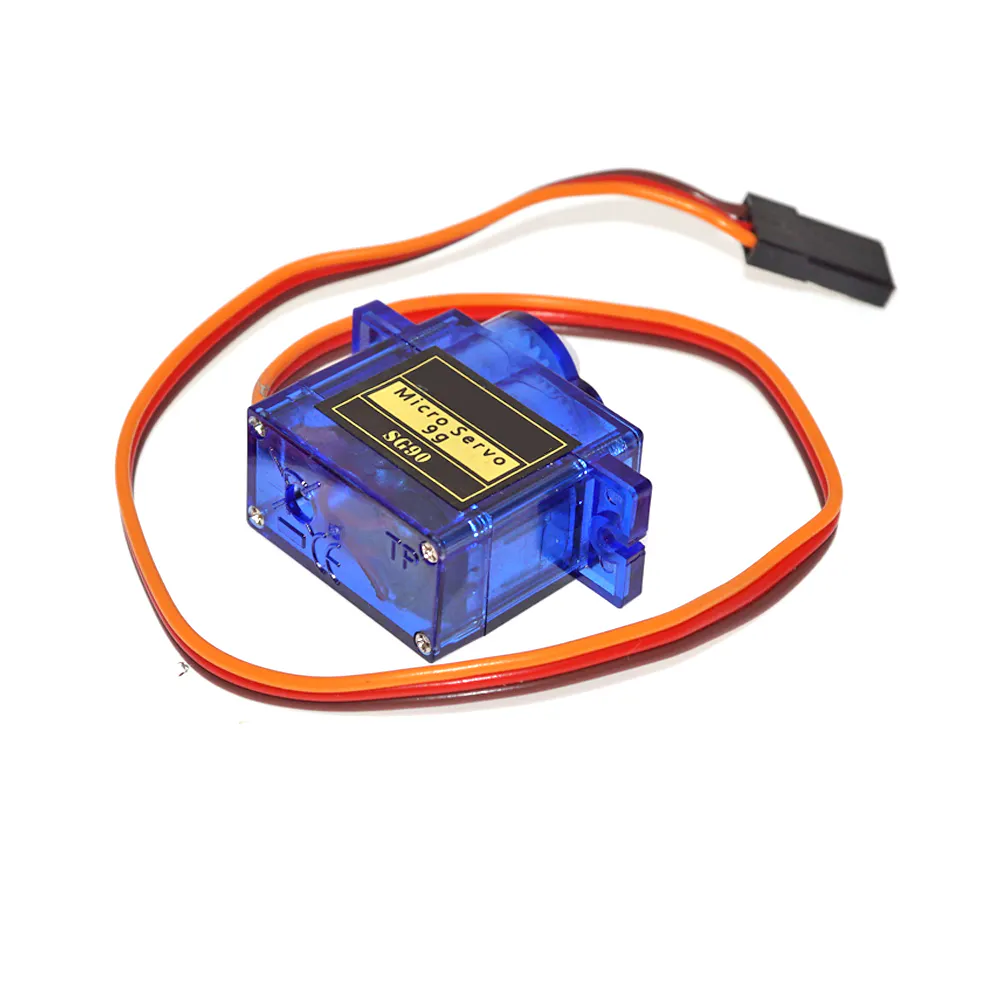SG90 Micro-servo motor
×1LED (generic)
×1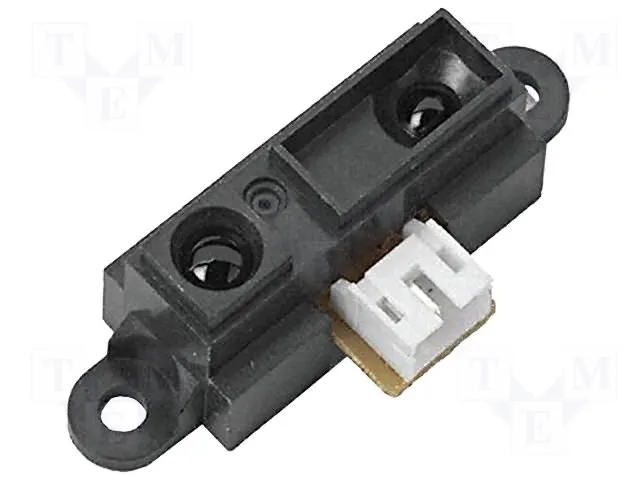Proximity Sensor
×3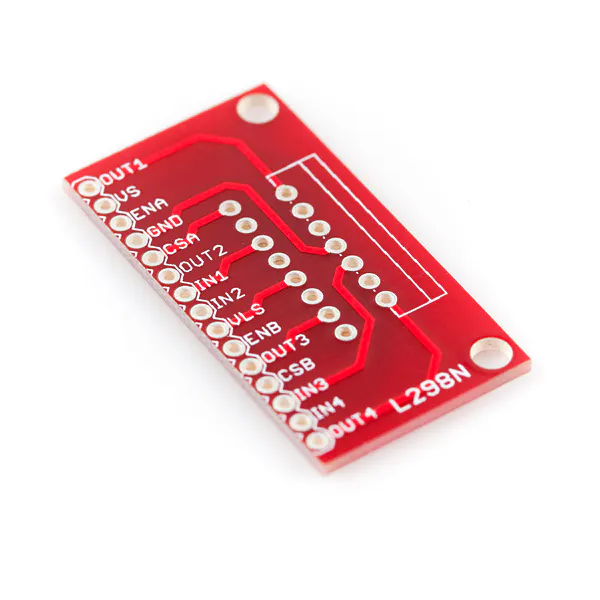SparkFun Full-Bridge Motor Driver Breakout - L298N
×1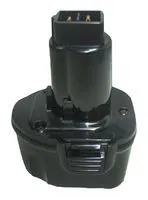Rechargeable Battery, 7.2 V
×1
 HC-05 Bluetooth
×2
 Gearmotor 120 rpm (generic)
×2
 Ball caster
×1

### Software apps and online servicesArduino IDE

### Hand tools and fabrication machines3D Printer (generic)

## Custom parts and enclosures

### 1.2 Printing and Assembling the Control Panel

STL and GCODE files of a security system from autonomous robot with SS-430 KEMET Proximity Sensor

### 2.2 Printing and Assembling the Autonomous Robot

STL and GCODE files of an autonomous microrobot

## Schematics

### 1. Open And Close the Gate

Electrical connection of the security system with SS-430 proximity sensor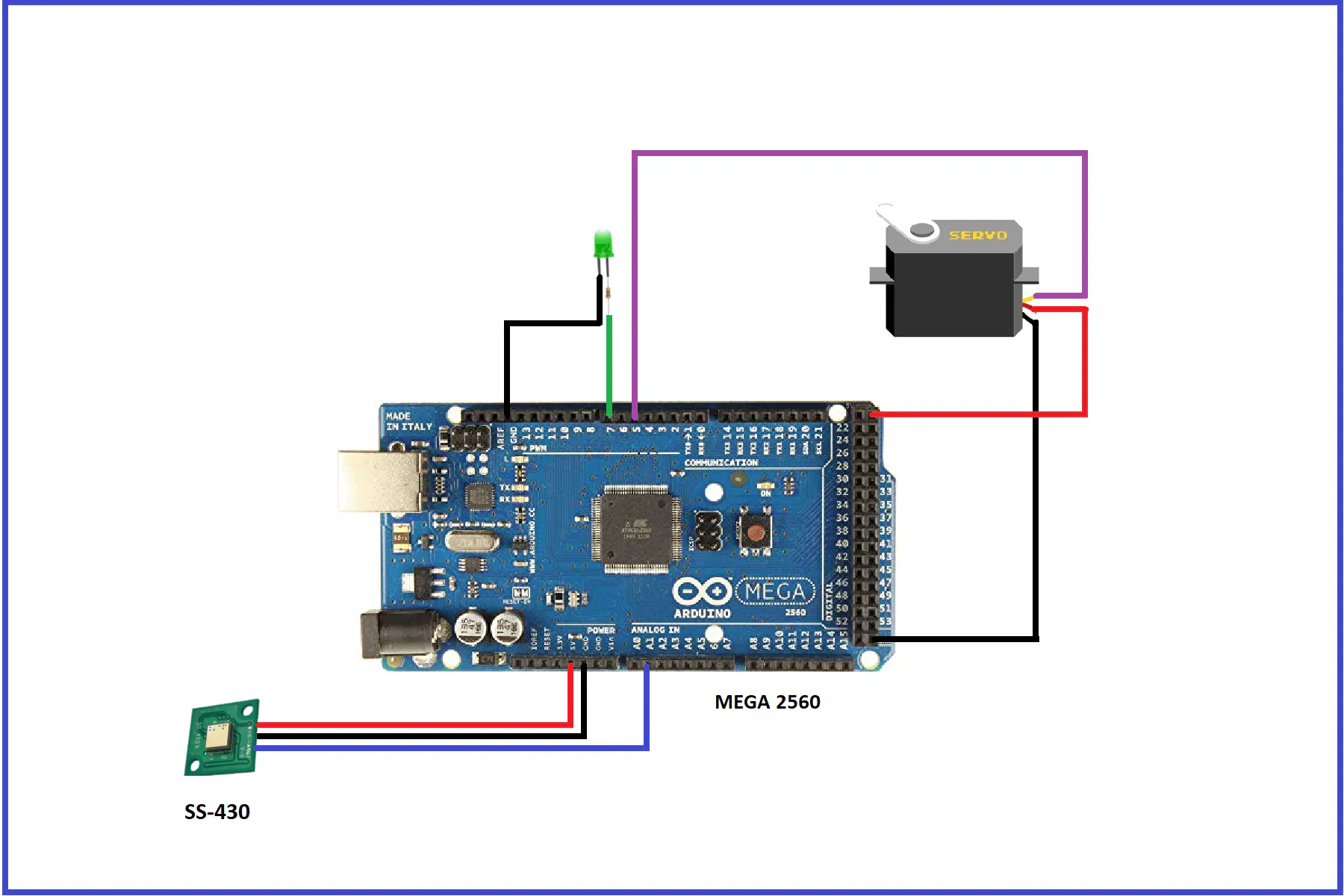### 2. Stop the Self-Driving Car - Transmitter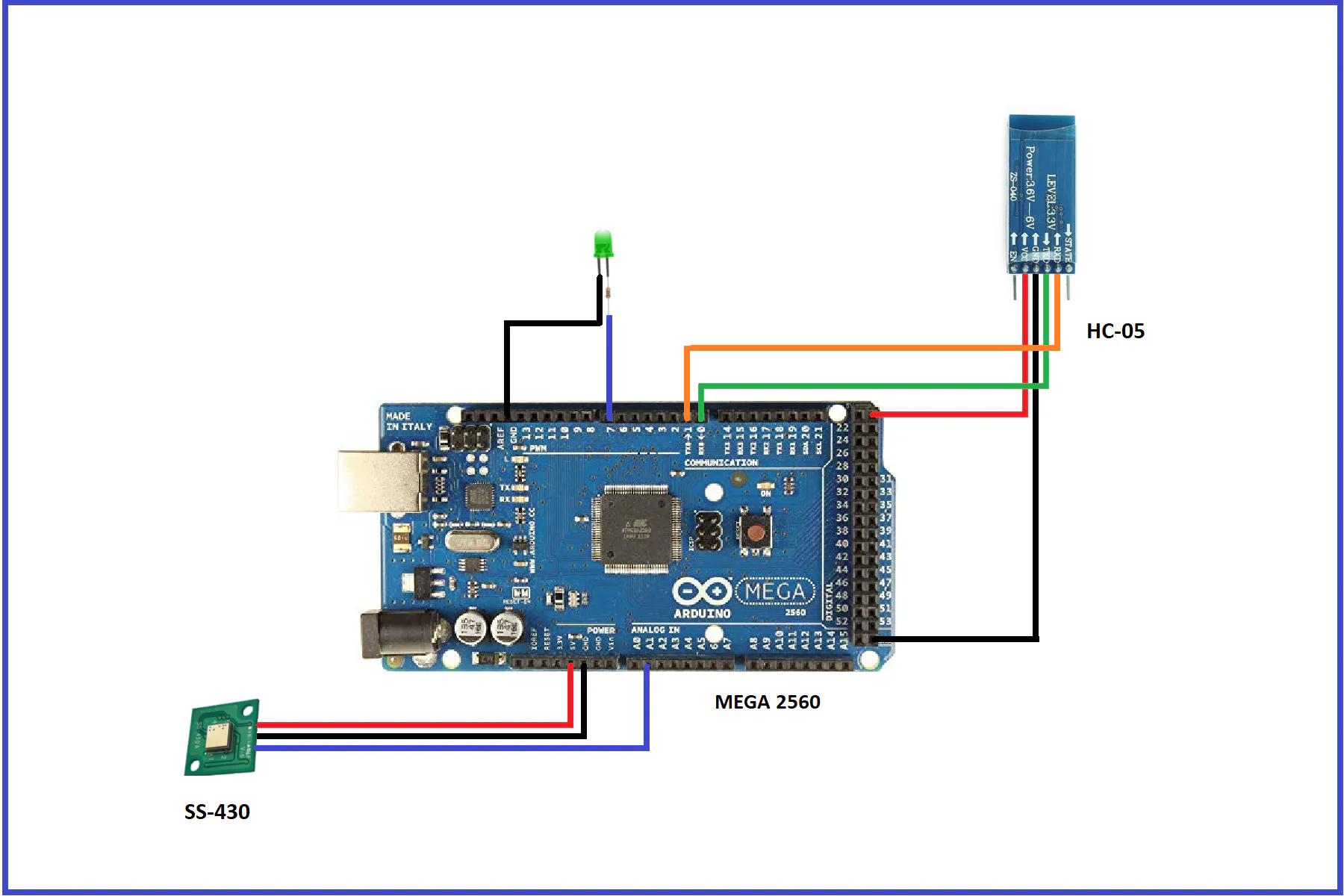### 2 Stop the Self-Driving Car - Receiver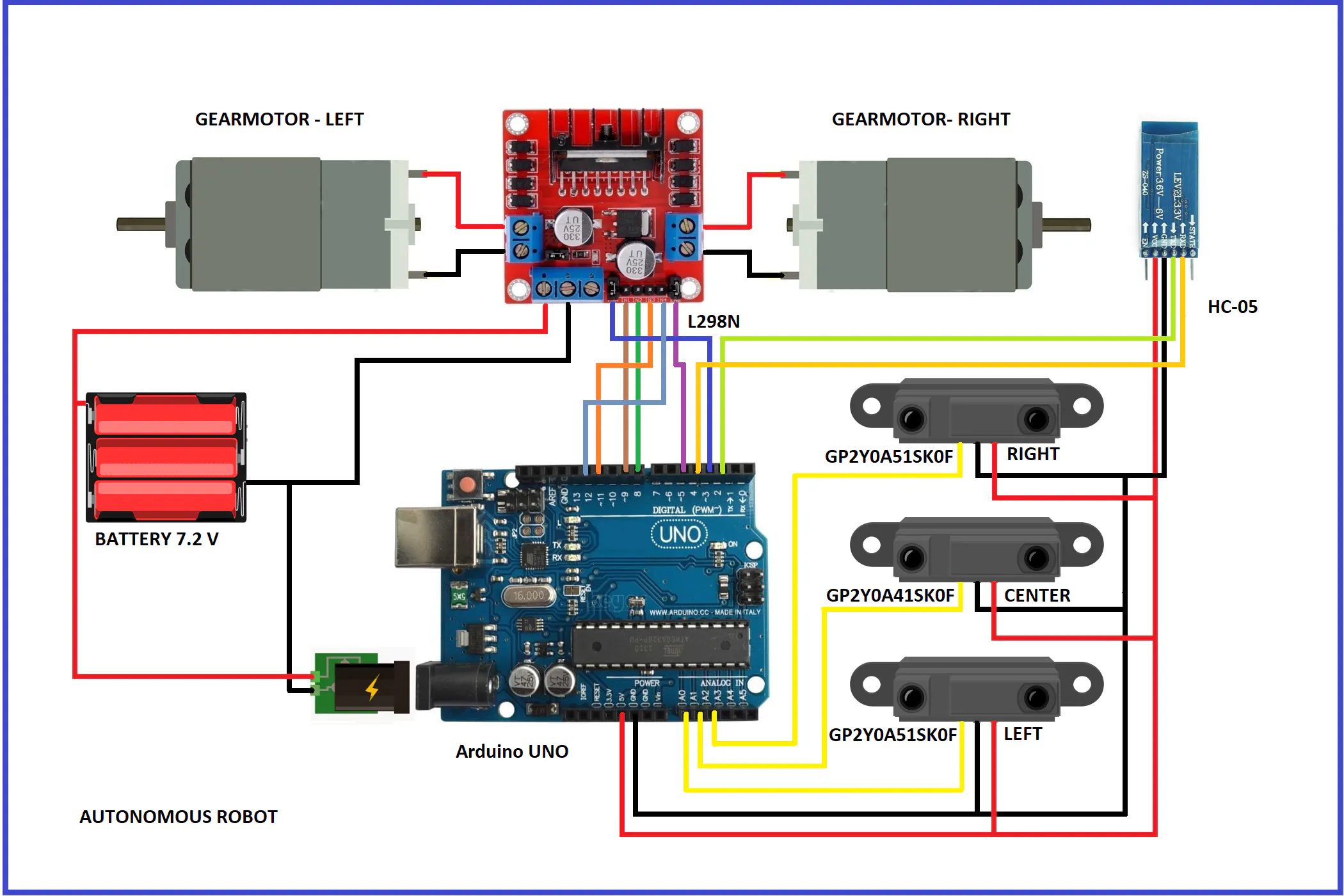## Code

### OpenAndCloseTheGate

Arduino
1. OPEN/CLOSE THE GATE
This code is part of the first section and must be uploaded to the Mega 2560 board
```// AUTHOR: GUILLERMO ALBERTO PEREZ GUILLEN

#include <Servo.h>

Servo myservo;  // create servo object to control a servo
int pos = 0;    // variable to store the servo position

// int Pyro = A1;
unsigned long IR_threshold = 199900;

// Note: SS-430 has two pulses of 200msec per detection.
// IR_threshold is in microsec (usec), therefore 199.9 msec threshold

int LED = 7;
int Detected = LOW;
int IR_sensed = 0;

void setup() {
myservo.attach(5);  // attaches the servo on pin 9 to the servo object
pinMode (7, OUTPUT); //LED Connected to Pin 7
pinMode (A1,INPUT); // IR Sensor connected to A1
}

void loop() {
while ((IR_sensed < 2)){ //Break after 2 good triggers
PyroRead = pulseIn(A1, HIGH); //Measure trigger point
if(PyroRead > IR_threshold){ //Make sure trigger is over 198msec)
IR_sensed++; //Mark as a good trigger
}
}

if (Detected == HIGH){ // Turn LED OFF if it was previous ON
Detected = LOW;
digitalWrite(7, LOW);
for (pos = 180; pos >= 0; pos -= 1) { // goes from 180 degrees to 0 degrees
myservo.write(pos);              // tell servo to go to position in variable 'pos'
delay(10);                       // waits 10ms for the servo to reach the position
}
}

else {
Detected = HIGH; // Turn LED ON if it was previous OFF
digitalWrite(7, HIGH);
for (pos = 0; pos <= 180; pos += 1) { // goes from 0 degrees to 180 degrees
// in steps of 1 degree
myservo.write(pos);              // tell servo to go to position in variable 'pos'
delay(10);                       // waits 10ms for the servo to reach the position
}
}
IR_sensed = 0;

delay(750); // Accept triggers after a 750 msec

}
```

### StopMotion

Arduino
2. STOP MOTION
This code is part of the second section and must be uploaded to the Mega 2560 board
```// AUTHOR: GUILLERMO ALBERTO PEREZ GUILLEN

unsigned long IR_threshold = 199900;

// Note: SS-430 has two pulses of 200msec per detection.
// IR_threshold is in microsec (usec), therefore 198msec threshold

int LED = 7;
int Detected = LOW;
int IR_sensed = 0;

void setup() {
Serial.begin(9600);
pinMode (7, OUTPUT); //LED Connected to Pin 7
pinMode (A1,INPUT); // IR Sensor connected to A1
}

void loop() {
while ((IR_sensed < 2)){ //Break after 2 good triggers
PyroRead = pulseIn(A1, HIGH); //Measure trigger point
if(PyroRead > IR_threshold){ //Make sure trigger is over 198msec)
IR_sensed++; //Mark as a good trigger
}
}

digitalWrite(7, HIGH);
Serial.println("a");  // stop the autonomous robot
delay(5000);
digitalWrite(7, LOW);
Serial.println(" ");  // stop the autonomous robot

IR_sensed = 0;
}
```

### AutonomousRobot

Arduino
2. STOP MOTION
This code is part of the second section and most be uploaded to de Arduino UNO board
```// AUTHOR: GUILLERMO ALBERTO PEREZ GUILLEN

#include <SoftwareSerial.h>

SoftwareSerial ModuloHC05 (2, 4);  // 2 is the pin RX ARDUINO - TX BT
// 4 is the pin TX ARDUINO - RX BT

//Motor A
int EN1 = 3; //Speed control - EN1
int IN1 = 9; //Direction - IN1
int IN2 = 8; //Direction - IN2

//Motor B
int EN2 = 5; //Speed control - EN2
int IN3 = 11; //Direction - IN3
int IN4 = 12; //Direction - IN4

int error=0;
int dif,difAnt=0;
const float Kp=0.1;
const float Kd=0.1;

int option;
int led = 13;

void setup() {
Serial.begin(9600);
ModuloHC05.begin(9600);
pinMode(led, OUTPUT);
pinMode(A0, INPUT); //left
pinMode(A1, INPUT); //forward
pinMode(A2, INPUT); //right
pinMode(EN1, OUTPUT);
pinMode(IN1, OUTPUT);
pinMode(IN2, OUTPUT);
pinMode(EN2, OUTPUT);
pinMode(IN3, OUTPUT);
pinMode(IN4, OUTPUT);
// Set initial rotation direction
digitalWrite(IN1, LOW);
digitalWrite(IN2, LOW);
digitalWrite(IN3, LOW);
digitalWrite(IN4, LOW);
digitalWrite(led, LOW);
}

void loop() {

char option;
float volts1 =  analogRead(A1)*0.0048828125;  // value from sensor * (5/1024)
float distance1 = 9*pow(volts1, -1); // worked out from datasheet graph //GP2Y0A41SK0F - 4 a 30 cm

error = floor(Kp*(dif)+Kd*(difAnt-dif));
difAnt=dif;
int d0 = constrain(255 - error, 0, 255);//left speed
int d1 = constrain(255 + error, 0, 255);//right speed

if (distance1 <= 10){
stop();
delay(100);

//backward 275 ms
{
digitalWrite(IN1, LOW);
digitalWrite(IN2, HIGH);
analogWrite(EN1, 128);  // average speed
digitalWrite(IN3, LOW);
digitalWrite(IN4, HIGH);
analogWrite(EN2, 128);  // average speed
}
delay(275);
stop();
delay(100);

//turn to the left 90 degrees
digitalWrite(IN1, HIGH);
digitalWrite(IN2, LOW);
analogWrite(EN1, 128);
digitalWrite(IN3, LOW);
digitalWrite(IN4, HIGH);
analogWrite(EN2, 128);
delay(480);
stop();
delay(100);
}
//move forward with calculated speeds
digitalWrite(IN1, HIGH);
digitalWrite(IN2, LOW);
analogWrite(EN1, d0);
digitalWrite(IN3, HIGH);
digitalWrite(IN4, LOW);
analogWrite(EN2, d1);
delay(50);

if (ModuloHC05.available()){
Serial.write(option);

if(option=='a') {
digitalWrite(led, HIGH);
stop();
delay(5000);
digitalWrite(led, LOW);
}
}
}

void stop(){
digitalWrite(IN1, LOW);
digitalWrite(IN2, LOW);
digitalWrite(IN3, LOW);
digitalWrite(IN4, LOW);
delay(10);
}
```

### Project repository: "Autonomous Robot Controlled with Pyro Sensors"

Folders: 1) AutonomousRobot; 2) OpenAndCloseTheGate; and 3) StopMotion

## Credits

### Guillermo Guillen

39 projects • 36 followers
Communications and Electronics Engineering, and Writer... "Don´t Expect Different Results If Your Habits Are The Same" A Einstein